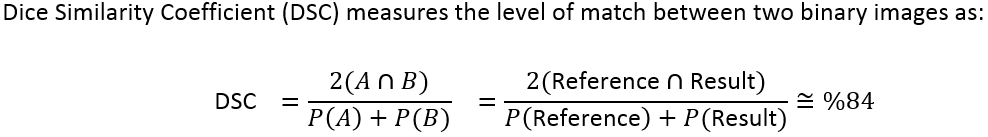# Calculate Dice Similarity Coefficient - Python

8539
2
12-19-2017 11:10 AMRegular Contributor

I'm trying to determine how to calculate the Dice Similarity Coefficient between two rasters. The one raster is the ground truth result of a road surface area, the second raster is the result from a Computer Vision and Machine Learning (Convolutional Neural Network). I'm trying to understand how the Dice Similarity Coefficient works so that I can replicate it as a Python\NumPy function to run against all the sample results and ground truth results that were manually determined.

NB: Id also like determine the following raster below which depicts True Positive; False Positive and False Negative

Wikipedia: Sorensen-Dice Cofficient

Formula:Raster Result:Tags (3)
2 RepliesbyMVP Esteemed Contributor

functionally equivalent I think... you will have SciPy installed if you are using recent versions of ArcMap or ArcGIS Pro.   So if memory serves, check to see if this is the same.

from scipy.spatial.distance import diceRegular Contributor

Thanks Dan, will check it out and get back to you.